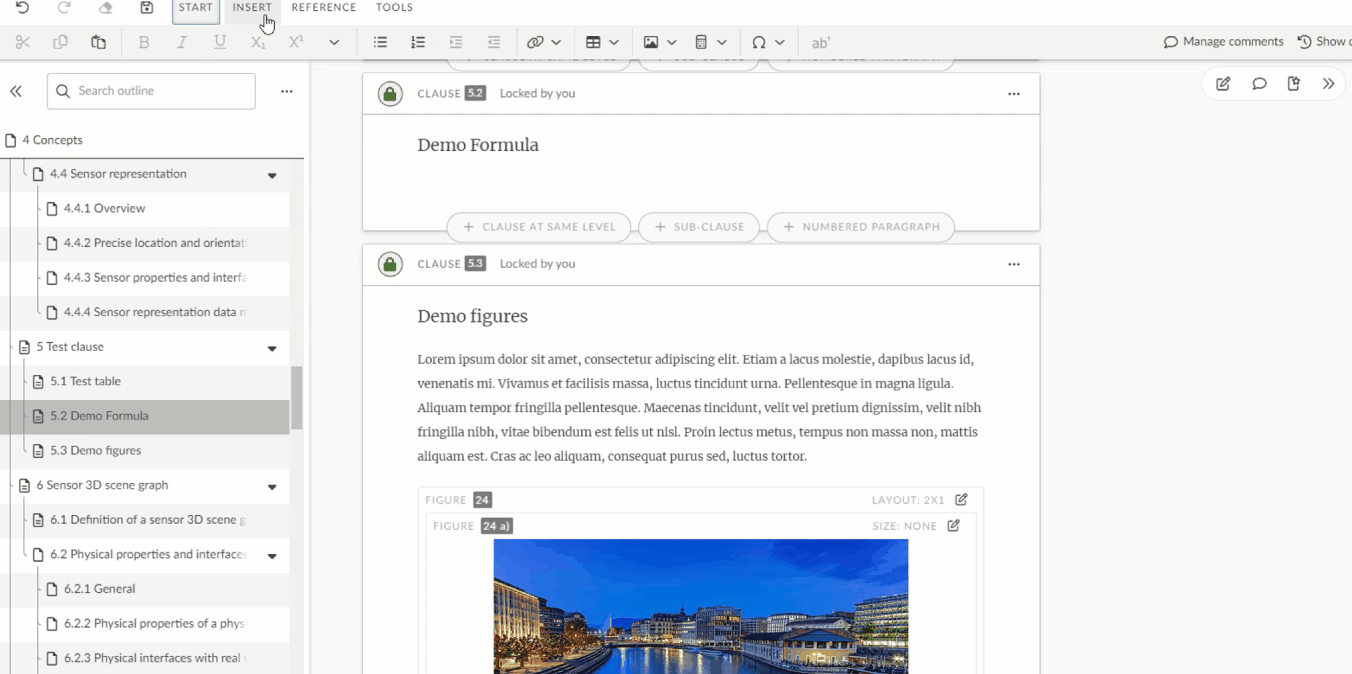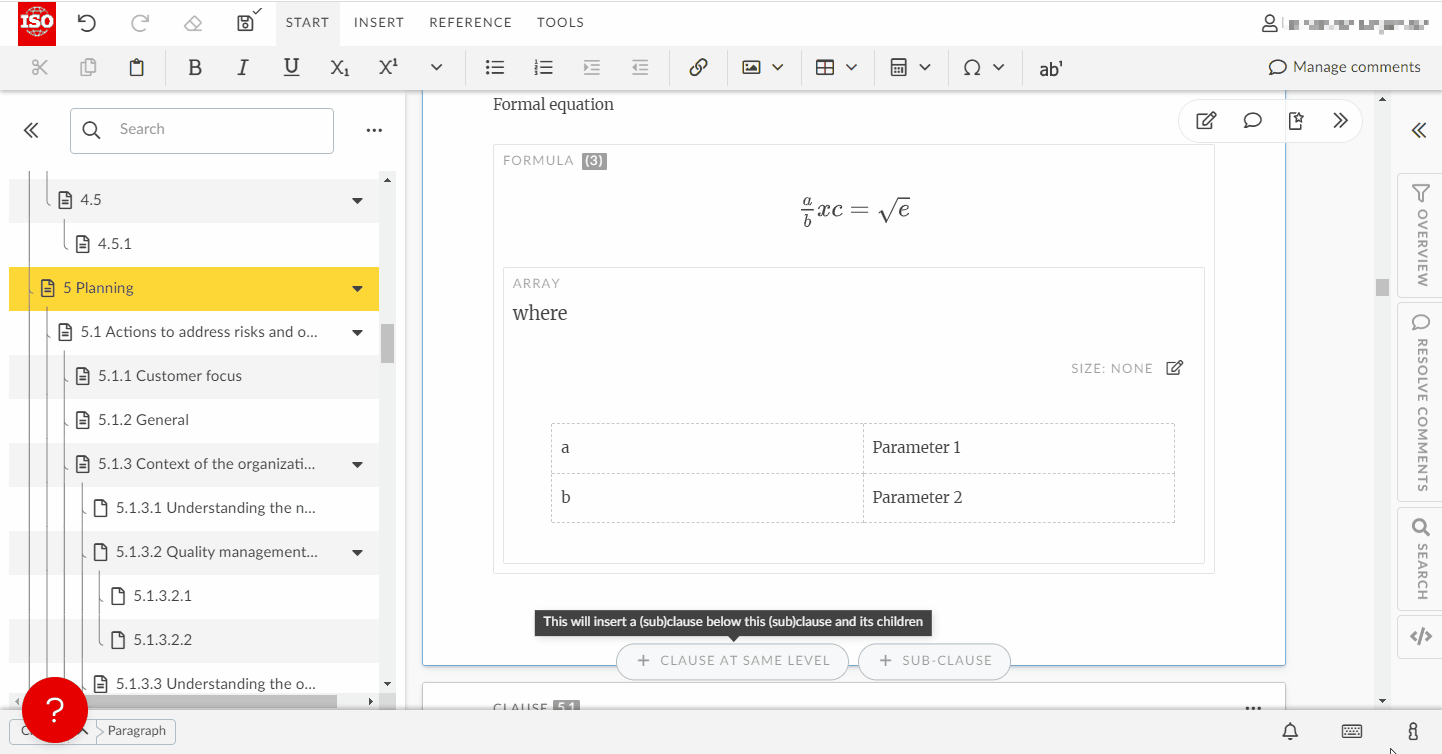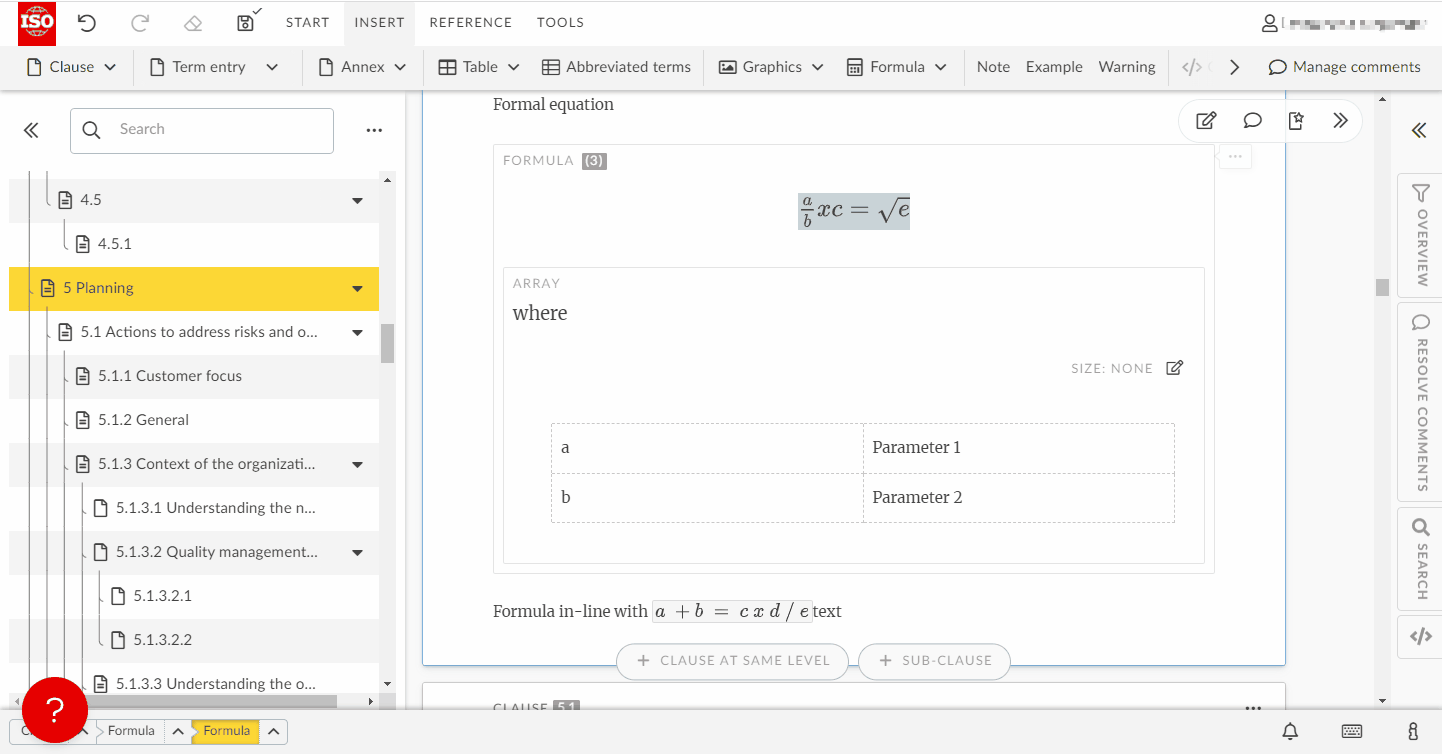Edit a formula

Authors

There are two types of formulas that can be added:

• Formal formulae: are numbered and can have a formula key
• Inline formulae: are not numbered and do not have a formula key

• To add a formula, put your cursor to the location where the formula should be inserted and click on Formula from the INSERT tab.
• A pop-up window with the equation editor will appear. Use the elements in the different tabs of the equation editor menu to enter your equation
• Then click Save to insert it into your document.
• To add a key to the equation, select Add Key from the element menu of the equation.• To add a mathematical expression in the flow of the text, i.e. an inline formula, place your cursor where you want to insert the equation.
• Go to the INSERT tab, click on Formula and Formula inline with text
• In the the formula editor that pops up, type your formula and click the Save button to insert the formula inline with your text:# Edit a formula

• You can edit your equation by right-clicking on it and selecting Edit Formula.
• You can also use the drawing tool to design your equations with the mouse or a pencil pad: